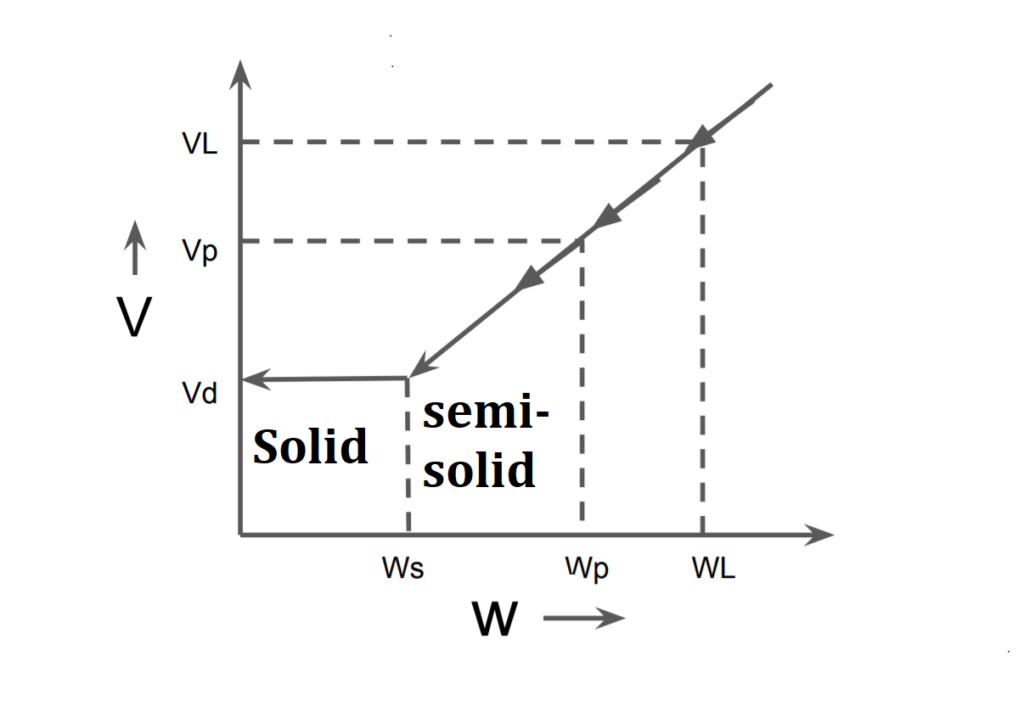# Shrinkage Limits- Consistency Atterberg Limits | Properties of Soil | Soil Mechanics

Contents

## Consistency Atterberg Limits

• Liquid Limit (WL)
• Plastic Limit (Wp)
• Shrinkage Limit (Ws)

## Shrinkage Limits(Ws)

• It is defined as the maximum water content at which reduction in the water content of soil does not lead to a reduction in the volume of soil, as below (Ws) replacement of water by air in equal volume takes place while reducing the water content.
• It may also be defined as the minimum water content at which soil is completely saturated.
• At shrinkage limit, soil passes semi-solid state of consistency to soil state of consistency & visa-versa.####which soil has more shrinkage limits, and Volume change (Compressibility) is less.

Note: Ws limit governs the compressibility of soil, the higher the shrinkage limit lower is compressibility (volume change).

## Determination of Shrinkage Limit

• Consider a sample of soil having water content more than shrinkage limit, let its mass & volume be M1,V1.
• The soil is subjected to drying as a result of which its volume decreases. At a particular stage of drying, when its water content is equal its shrinkage limit, it mass & volume be M2,V2 on complete drying let its mass of volume be Md &Vd.
######we don’t know about stage.2 that water content is equal to shrinkage limit or not . But we know the volume of soil at shrinkage limit is equal to the volume of soil at dry state. so stage 2 is only calculation purpose.

V2=Vd

V2≠Vs

Vd≠Vs

Md=Ms

Mass of water in stage 1= M1-Md.

Loss of water from stage 1 to 2= (V1-V2)$$ρ_w$$=(V1-Vd)$$ρ_w$$.

Mass of water in stage 2= Mass of water in stage 1  –  Loss of water from stage 1 to 2.

Mass of water in stage 2=(M1-Md) – (V1-Vd)$$ρ_w$$.

Shrinkage limit Ws$$=\frac{\text { Mass of water in stage 2 }}{\text { Mass of solids}}$$.

$$W_s=\frac{(M_1-Md)-(V1-Vd)ρ_w}{Md}$$.

$$W_s=\frac{(M_1-Md)}{Md}-\frac{(V1-Vd)}{Md}$$.

$$W_s=w_1-\frac{(V1-Vd)}{Md}$$.

 Subject Soil Mechanics Unit Soil Formation & Properties of Soil Topic Shrinkage Limits- Consistency Atterberg Limits Next Topic Shrinkage Index, Shrinkage Ratio, Volumetric shrinkage, Linear Shrinkage, Plasticity Index Previous Topic Plastic Limits- Consistency Atterberg Limits

error: Content is protected !!
Scroll to Top